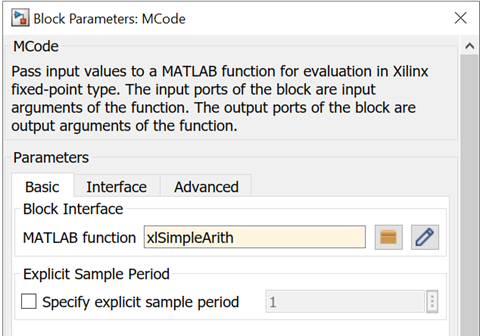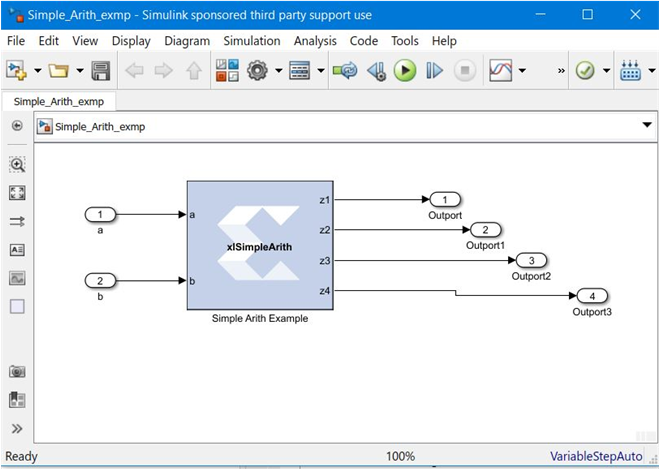# Simple Arithmetic Operations - 2022.1 English

## Vitis Model Composer User Guide (UG1483)

Document ID
UG1483
Release Date
2022-05-26
Version
2022.1 English

This example shows some simple arithmetic operations and type conversions. The following shows the xlSimpleArith.m file, which specifies the `xlSimpleArith` M-function.

``````function [z1, z2, z3, z4] = xlSimpleArith(a, b)
% xlSimpleArith demonstrates some of the arithmetic operations
% supported by the Xilinx MCode block. The function uses xfix()
% to create Xilinx fixed-point numbers with appropriate
% container types.%
% You must use a xfix() to specify type, number of bits, and
% binary point position to convert floating point values to
% Xilinx fixed-point constants or variables.
% By default, the xfix call uses xlTruncate
% and xlWrap for quantization and overflow modes.
% const1 is Ufix_8_3
const1 = xfix({xlUnsigned, 8, 3}, 1.53);
% const2 is Fix_10_4
const2 = xfix({xlSigned, 10, 4, xlRound, xlWrap}, 5.687);
z1 = a + const1;
z2 = -b - const2;
z3 = z1 - z2;
% convert z3 to Fix_12_8 with saturation for overflow
z3 = xfix({xlSigned, 12, 8, xlTruncate, xlSaturate}, z3);
% z4 is true if both inputs are positive
z4 = a>const1 & b>-1;
``````

This M-function uses addition and subtraction operators. The MCode block calculates these operations in full precision, which means the output precision is sufficient to carry out the operation without losing information.

One thing worth discussing is the `xfix` function call. The function requires two arguments: the first for fixed-point data type precision and the second indicating the value. The precision is specified in a cell array. The first element of the precision cell array is the type value. It can be one of three different types: `xlUnsigned`, `xlSigned`, or `xlBoolean`. The second element is the number of bits of the fixed-point number. The third is the binary point position. If the element is `xlBoolean`, there is no need to specify the number of bits and binary point position. The number of bits and binary point position must be specified in pair. The fourth element is the quantization mode and the fifth element is the overflow mode. The quantization mode can be one of `xlTruncate`, `xlRound`, or `xlRoundBanker`. The overflow mode can be one of `xlWrap`, `xlSaturate`, or `xlThrowOverflow`. Quanitization mode and overflow mode must be specified as a pair. If the quantization-overflow mode pair is not specified, the `xfix` function uses `xlTruncate` and `xlWrap` for signed and unsigned numbers. The second argument of the `xfix` function can be either a double or a Xilinx fixed-point number. If a constant is an integer number, there is no need to use the `xfix` function. The Mcode block converts it to the appropriate fixed-point number automatically.

After setting the dialog box parameter MATLAB function to `xlSimpleArith`, the block shows two input ports a and b, and four output ports z1, z2, z3, and z4.

Figure 1. xlSimpleArith MCode ParameterFigure 2. xlSimpleArith DesignM-functions using Xilinx data types and functions can be tested in the MATLAB Command Window. For example, if you type: ```[z1, z2, z3, z4] = xlSimpleArith(2, 3)``` in the MATLAB Command Window, you'll get the following lines:

``````UFix(9, 3): 3.500000
Fix(12, 4): -8.687500
Fix(12, 8): 7.996094
Bool: true
``````

Notice that the two integer arguments (2 and 3) are converted to fixed-point numbers automatically. If you have a floating-point number as an argument, an `xfix` call is required.